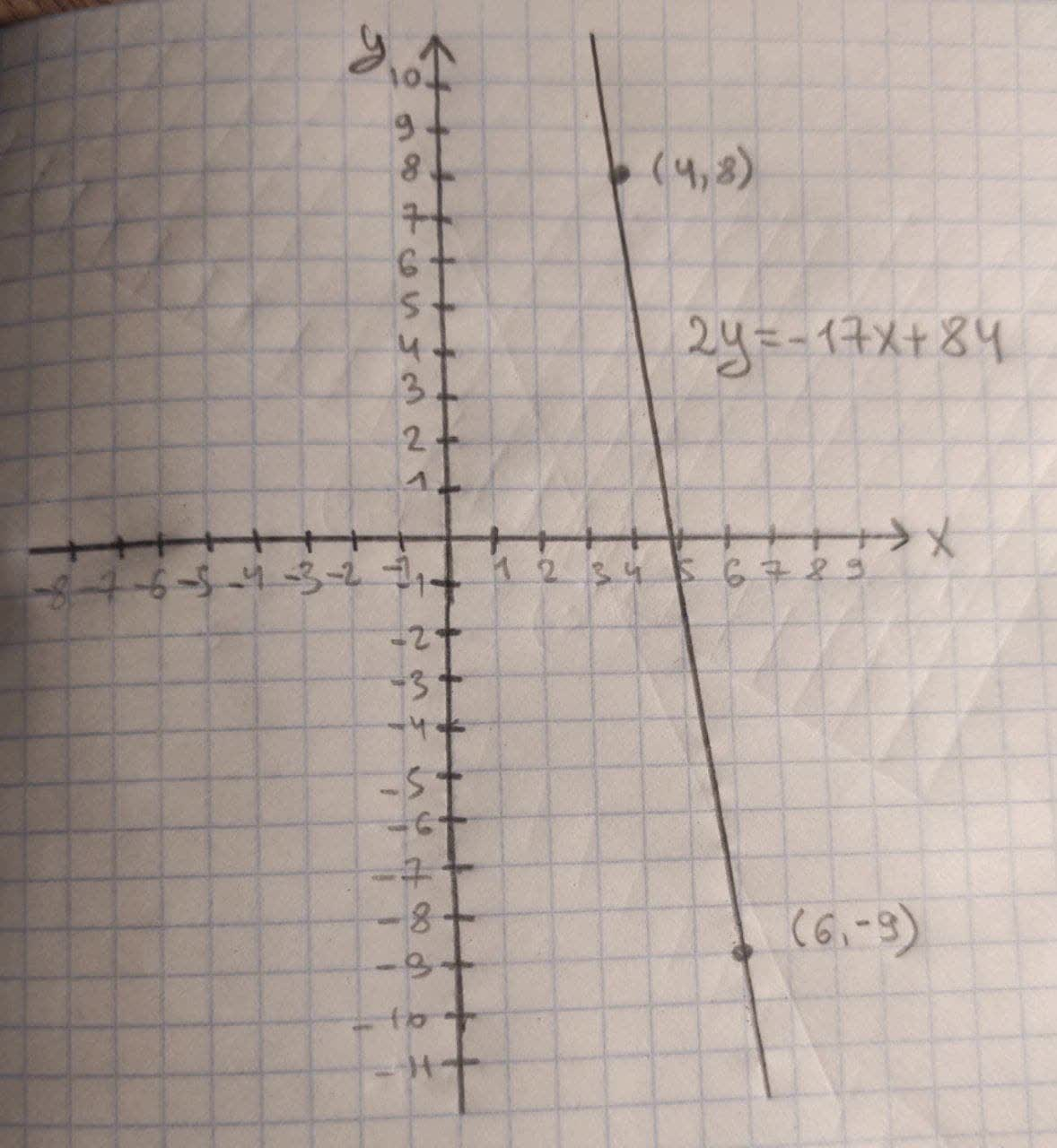# What would be the linear equation for this function, & the graph?(X_1,Y_1)=(4,8), (X_2,Y_2)=(6,-9)Wribreeminsl 2020-10-23 Answered

What would be the linear equation for this function, and the graph?
$\left({X}_{1},{Y}_{1}\right)=\left(4,8\right),\left({X}_{2},{Y}_{2}\right)=\left(6,-9\right)$

You can still ask an expert for help

• Questions are typically answered in as fast as 30 minutes

Solve your problem for the price of one coffee

• Math expert for every subject
• Pay only if we can solve itSadie Eaton
Formula used:
Point-slope form of line:
$\left({y}_{2}-{y}_{1}\right)=m\left({x}_{2}-{x}_{1}\right)$
So, by the Point-Slope form of line the equation of line is given below:
$\left(-9-8\right)=m\left(6-4\right)$
$-17=m×2$
$2m=-17$
$m=-\frac{17}{2}$
Now $m=-\frac{17}{2}$ and take any points to find the linear equation.
$\left({x}_{1},{y}_{1}\right)=\left(4,8\right)\phantom{\rule{1em}{0ex}}\text{and}\phantom{\rule{1em}{0ex}}m=-\frac{17}{2}$
$\left(y-{y}_{1}\right)=m\left(x-{x}_{1}\right)$
$\left(y-8\right)=-\frac{17}{2}\left(x-4\right)$
$\left(y-8\right)=\frac{-17x}{2}+34$
$y=\frac{-17x}{2}+34+8$
$y=\frac{-17x}{2}+42$
$y=\frac{-17x+84}{2}$
$2y=-17{x}_{84}$
So, the graph is given below: